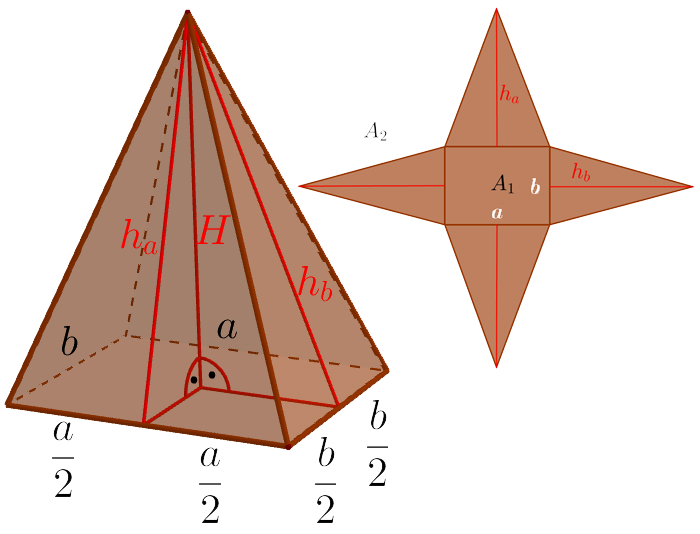# PyramideArea

$A={A}_{1}+{A}_{2}=ab+a{h}_{a}+b{h}_{b}$

Base Area

${A}_{1}=a·b$

Side Area

${A}_{2}=\frac{2a{h}_{a}}{2}+\frac{2b{h}_{b}}{2}=a{h}_{a}+b{h}_{b}$

Side Triangles

${h}_{a}=\sqrt{{\left(\frac{b}{2}\right)}^{2}+{H}^{2}}$
${h}_{b}=\sqrt{{\left(\frac{a}{2}\right)}^{2}+{H}^{2}}$

Volume

$V=\frac{{A}_{1}·H}{3}=\frac{abH}{3}$
Keywords: pyramide, area, volume